# Two squares

Two squares whose sides are in the ratio 5:2 have sum of its perimeters 73 cm. Calculate the sum of area this two squares.

Correct result:

S1+S2 =  197.1 cm2

#### Solution: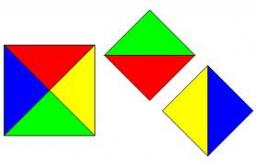Our examples were largely sent or created by pupils and students themselves. Therefore, we would be pleased if you could send us any errors you found, spelling mistakes, or rephasing the example. Thank you!

Please write to us with your comment on the math problem or ask something. Thank you for helping each other - students, teachers, parents, and problem authors.Tips to related online calculators
Check out our ratio calculator.
Do you have a linear equation or system of equations and looking for its solution? Or do you have quadratic equation?

#### You need to know the following knowledge to solve this word math problem:

We encourage you to watch this tutorial video on this math problem:

## Next similar math problems:

• The playgroundThe playground has the shape of a square with a side of 64 m. It is fenced on three sides. What is the area of the playground and how long is its fence?
• Net of a cube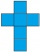This net can be folded to form a cube with a side length of 20 units. What is the surface area of the cube in square units?
• Carpet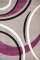How many crowns CZK do we pay for a carpet for a bedroom, when 1m of square carpet costs 350 CZK and the bedroom has dimensions of 4m and 6m? How many crowns do we pay for a strip around the carpet, when 1m of the strip costs 15 CZK?
• Two sides paintThe door has the shape of a rectangle with dimensions of 260cm and 170cm. How many cans of paint will be needed to paint this door if one can of paint cover 2m2 of the area? We paint the doors on both sides.
• Chess competition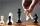4 chess players took part in the competition. How many tournaments have taken place if every chess player has fought everyone once?
• Average priceSixth grade went on a trip to Moravia. Each of the 26 pupils paid CZK 320 and the school paid a total of CZK 3,510. What was the average price of a trip per student?
• The temperature 8The temperature on Monday was –6°C. On Tuesday the temperature was 3 degrees lower. Write down the temperature on Tuesday.
• Cyclist 12What is the average speed of a cycle traveling at 20 km in 60 minutes in km/h?
• My fatherMy father cut 78 slats on the fence. The shortest of them was 97 cm long, the longer one was 102 cm long. What was the total length of the slats in cm?
• Equation with one variableSolve the following equation with one unknown: 5(7s + 5) =130
• The swallow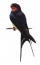The swallow will fly 2.8 km per minute. How many km will the swallow fly in one hour?
• Storm 3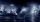If the temperature yesterday was 56 and today is 13 degrees cooler, what is today's temperature?
• The temperature 6The temperature was 47°F on Thursday and 60°F on Friday. How much did the temperature rise?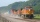The whole loaded train weighs 360 tons. It has twenty wagons, each carrying 12 tons of grain. What is the weight of the locomotive?If x=2, y=-5 and z=3 what is the value of x-2y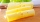From 40 liters of milk is produced 8 kg of cheese how many liters of milk are needed to produce 2 kg of cheese?Ski organizers should print the start numbers from 1 to 45. How many times will they use the number 3 when printing?